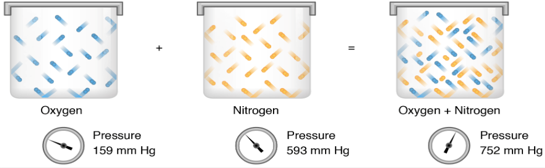# Dalton's Law Formula

## Dalton's Law Formulas - Dalton's Law of Partial Pressure

This is an empirical law which states that the total pressure exerted by a mixture of non-reacting gases is equal to the sum of partial pressures of individual gases.Note: It is important to have non-reacting gases as the pressure is dependent upon the number of moles of gas taken in the mixture. If the gases react, the number of moles may change and the total pressure will be not be equal to sum of the partial pressure of gases we took initially.

Mathematically,

Ptotal = P1 + P2 + P3 + ….. + Pn

Where ‘Ptotal' is the total pressure of the mixture, and ‘P1, P2, P3 … Pn are the partial pressures exerted by individual gases.

Example: A container contains three gases, N2, O2 and Ar, with partial pressures of 23.3 kPa, 40.9 kPa and 13.7 kPa respectively. What is the total pressure inside the container?
Solution:
PN2 = 23.3 kPa; PO2 = 40.9 kPa; PAr = 13.7 kPa
PT = ?
PT = PN2 + PO2 + PAr
PT = 23.3 kPa + 40.9 kPa + 13.7 kPa = 77.9 kPa

Question: In the chem lab, a student filled a balloon with 2 different gases, CO2 and N2. The atmospheric pressure in the chem lab was 770.0 mmHg and the partial pressure of CO2 added to the balloon was 0.3255 atm. What was the partial pressure of N2 in the balloon?
Options:
(a) 522.6 mmHg
(b) 540.6 mmHg
(c) 640.6 mmHg
(d) 840.7 mmHg